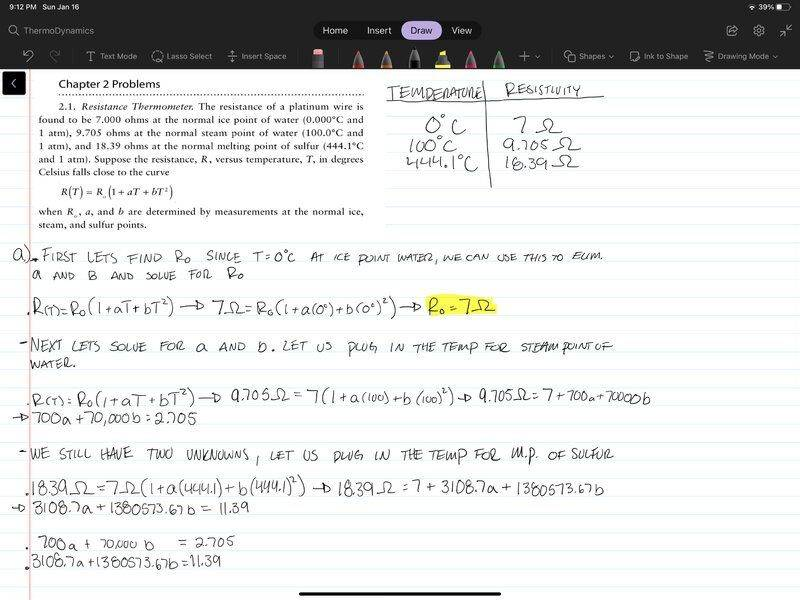# Finding the coefficients of a Resistance vs. Temp curve

guyvsdcsniper
Homework Statement:
Find Ro, a, and b.
Relevant Equations:
R(T) = Ro (1 + aT + bT2 )
I am asked to find Ro, a, and b. Th problem states the values are determined by the measurements at the normal ice, steam and sulfur points. So I approached the problem by plugging the the temperature problems. For 0°C, Ro reduces to 7 ohms. Then for the other two non zero temperatures, it looks like I am left with a system of equations.

I am a bit stumbled because the values associated for a and b of both equations arent factors of each other, so canceling out seems a bit trickier. I don't really recall running across a problem like this before.

Am I approaching this problem correctly? If so what is a way to solve for a and b?Homework Helper
Gold Member
You have three temperatures which means that you can write three equations, one at each temperature, and three unknowns. The first equation gives you (as you have already found) R0 = 7 Ω. Use that value in the other two equations which form a system of 2 equations and 2 unknowns a and b. Can you solve that?

guyvsdcsniper
You have three temperatures which means that you can write three equations, one at each temperature, and three unknowns. The first equation gives you (as you have already found) R0 = 7 Ω. Use that value in the other two equations which form a system of 2 equations and 2 unknowns a and b. Can you solve that?
I figured it out. I actually just finished a linear algebra course and they never gave us a problem like that where the coefficients weren't perfect factors of each other.

Very noob post. Sorry lol

•